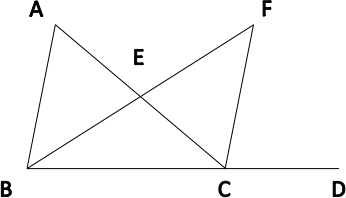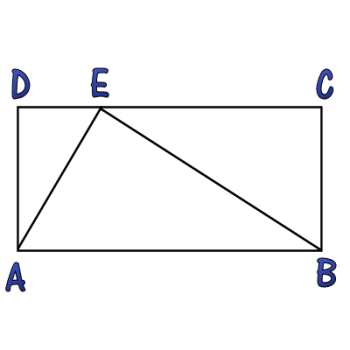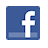Games
Problems
Go Pro!

# Angle Riddle

Pro Problems > Math > Geometry > Triangles

## Angle Riddle

The angles of a triangle meet the following criteria:

• All the angle measures are prime numbers.
• All the angle measures are distinct.
• Exactly two of my angle measures are palindromic.
• The difference between my two largest angle measures is a two digit number x.
• One of the digits of x is twice the other digit.
Presentation mode
Problem by MrMath

## Solution

In order to make it feasible for teachers to use these problems in their classwork, no solutions are publicly visible, so students cannot simply look up the answers. If you would like to view the solutions to these problems, you must have a Virtual Classroom subscription.Assign this problem
Click here to assign this problem to your students.

## Similar Problems

### Angle Bisector

In the diagram shown,m ∠DCF = 80
m ∠ACF = 30
m ∠FBD = 50.

If segment BF bisects  ∠ABD, find m ∠BAC.

### Three Triangles

The angles in a triangle have measures x, x + 10, and y.

The angles in a second triangle have measures x + y, 40, and 50.

The angles in a third triangle have measures x – y, 40, and z.

What is the value of z?

### Triangle in a Square

Triangle ABC is inside square ABDE, and C lies along side DE. If the area of the triangle is 24 square units, what is the length of AB?

### Triangle and RectangleIn the diagram displayed, the triangle splits the rectangle into three similar triangles. Find the value of AD2 divided by the product of DE and EC.

### Three Two-Digit Angle Measures

The measures of the angles in a triangle are all two digit numbers, and two of them end in 3. Exactly two of them contain the digit 4. None of the angle measures are multiples of three. What are the measures of the angles?

### Triangle Variables

An isosceles triangle is a triangle in which two of the angles have the same measure. In an isosceles triangle, the angle measures are 30 + x, 62 - x, and 10 + 2x + y. What are the possible numeric values for the measures of the angles?

### Two Triangles with Angle Measures

In a triangle, the angles measures are: 3x + 44, x, and 2x - 20. In a second triangle, two of the angle measures are x + 20 and 2x - 5. What is the measure of the third angle?

### Forty-five and Thirty

Two of the angles of a triangle are 45 degrees and 30 degrees.  The altitude from the third angle has length 15 units. What is the triangle's area?

### Quadratic Triangle

The angles in a triangle have measures x2 - 5, 2x + 18, and x + 37. What is the measure of the largest angle in the triangle?

### Angle Measure

Line segment AB is intersected by segment DC, with D on AB, between A and B.

In triangle ADC, m∠ADC = 30º + x and  m∠DAC = 50º

In triangle ABC, m∠ACB = 115º + x

In triangle CDB, m∠CDB = 140º + x

Find m∠CBD.

Arithmetic Angles, Split Triangle, Triangle in a Square, Angles in a Triangle

# Featured Games on This Site

Match color, font, and letter in this strategy game
Trap all the dots in this problem-solving puzzle

# Blogs on This SiteReviews and book lists - books we love!The site administrator fields questions from visitors.Like us on Facebook to get updates about new resources